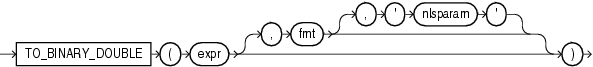TO_BINARY_DOUBLE

SyntaxDescription of the illustration to_binary_double.gif

Purpose

TO_BINARY_DOUBLE returns a double-precision floating-point number.

• expr can be a character string or a numeric value of type NUMBER, BINARY_FLOAT, or BINARY_DOUBLE. If expr is BINARY_DOUBLE, then the function returns expr.

• The optional 'fmt' and 'nlsparam' arguments are valid only if expr is a character string. They serve the same purpose as for the TO_CHAR (number) function.

• The case-insensitive string 'INF' is converted to positive infinity.

• The case-insensitive string '-INF' is converted to negative identity.

• The case-insensitive string 'NaN' is converted to NaN (not a number).

You cannot use a floating-point number format element (F, f, D, or d) in a character string expr.

Conversions from character strings or NUMBER to BINARY_DOUBLE can be inexact, because the NUMBER and character types use decimal precision to represent the numeric value, and BINARY_DOUBLE uses binary precision.

Conversions from BINARY_FLOAT to BINARY_DOUBLE are exact.

Examples

The examples that follow are based on a table with three columns, each with a different numeric data type:

CREATE TABLE float_point_demo
(dec_num NUMBER(10,2), bin_double BINARY_DOUBLE, bin_float BINARY_FLOAT);

INSERT INTO float_point_demo
VALUES (1234.56,1234.56,1234.56);

SELECT * FROM float_point_demo;

DEC_NUM BIN_DOUBLE  BIN_FLOAT
---------- ---------- ----------
1234.56 1.235E+003 1.235E+003

The following example converts a value of data type NUMBER to a value of data type BINARY_DOUBLE:

SELECT dec_num, TO_BINARY_DOUBLE(dec_num)
FROM float_point_demo;

DEC_NUM TO_BINARY_DOUBLE(DEC_NUM)
---------- -------------------------
1234.56                1.235E+003

The following example compares extracted dump information from the dec_num and bin_double columns:

SELECT DUMP(dec_num) "Decimal",
DUMP(bin_double) "Double"
FROM float_point_demo;

Decimal                     Double
--------------------------- ---------------------------------------------
Typ=2 Len=4: 194,13,35,57   Typ=101 Len=8: 192,147,74,61,112,163,215,10